Formulas, as the name indicates are calculations that you could define in ManageEngine Analytics Plus to help you create the required reports. ManageEngine Analytics Plus provides a powerful formula engine to create any type of calculations required, to enable creating the required reports. Formulas are mathematical expressions that you create, which contains any combination of numbers, references of table columns, operators and functions etc. Functions are predefined mathematical formulas which are designed to perform specific well-known calculations.

ManageEngine Analytics Plus supports different flavors of formulas. The following is a summary of the various types of formulas that are supported in ManageEngine Analytics Plus:

• Custom Formula Columns: These are formula types where you could add a new column to your data table, whose values are derived based on the calculation/formula defined. You can define any powerful mathematical formula which uses the table columns in combination with operators and in-built functions. As the output of the formula adds a new column in the table is called a Formula Column.

• In-built Formulas: ManageEngine Analytics Plus provides a useful set of in-built custom formulas, available by default, to perform common calculations easily. These formulas can be applied only to single column. The result of a calculation (formula) will be added as another column to the base table.
• Aggregate Formulas: Aggregate Formulas are formulas where you should use aggregate functions (SUM, AVG..) in the calculation. The result of Aggregate Formulas will not be added as another column in the base table. They will just be associated to the table on which it has been created and can be used in creating reports (Charts, Pivot Tables and Summary Views) like any other table columns.

## Custom Formula

ManageEngine Analytics Plus allows you to define your own powerful formulas to meet your specific reporting requirements. This enables you to easily perform from simple calculations such as addition and subtraction to a complex combination of in-built functions. As the output of the formula adds a new column in the table is called a Formula Column. You can use these formula columns in creating reports in the same way you use other columns in a table.

ManageEngine Analytics Plus provides you with a variety of in-built functions which are predefined mathematical formulas which are designed to perform specific well-known calculations easily. These in-built functions can be used in combination with the table columns and basic arithmetic operators like +, -, / and * to create your formula column.

To create and use custom formula columns:

• From the Explorer tab of the workspace, select the table to which you want to add the formula column.

• Select Add > Formula Column option from the toolbar or select a column in the table and choose Formula Column from the right click pop-up menu.

• The Formula Column dialog box will open as shown below. This dialog box provides easy access to the names of the columns in your table, as well as to many of the in-built functions that are available to create formulas. A brief description of the function with syntax and an example will be displayed at the bottom of the dialog box when you hover your mouse over a function.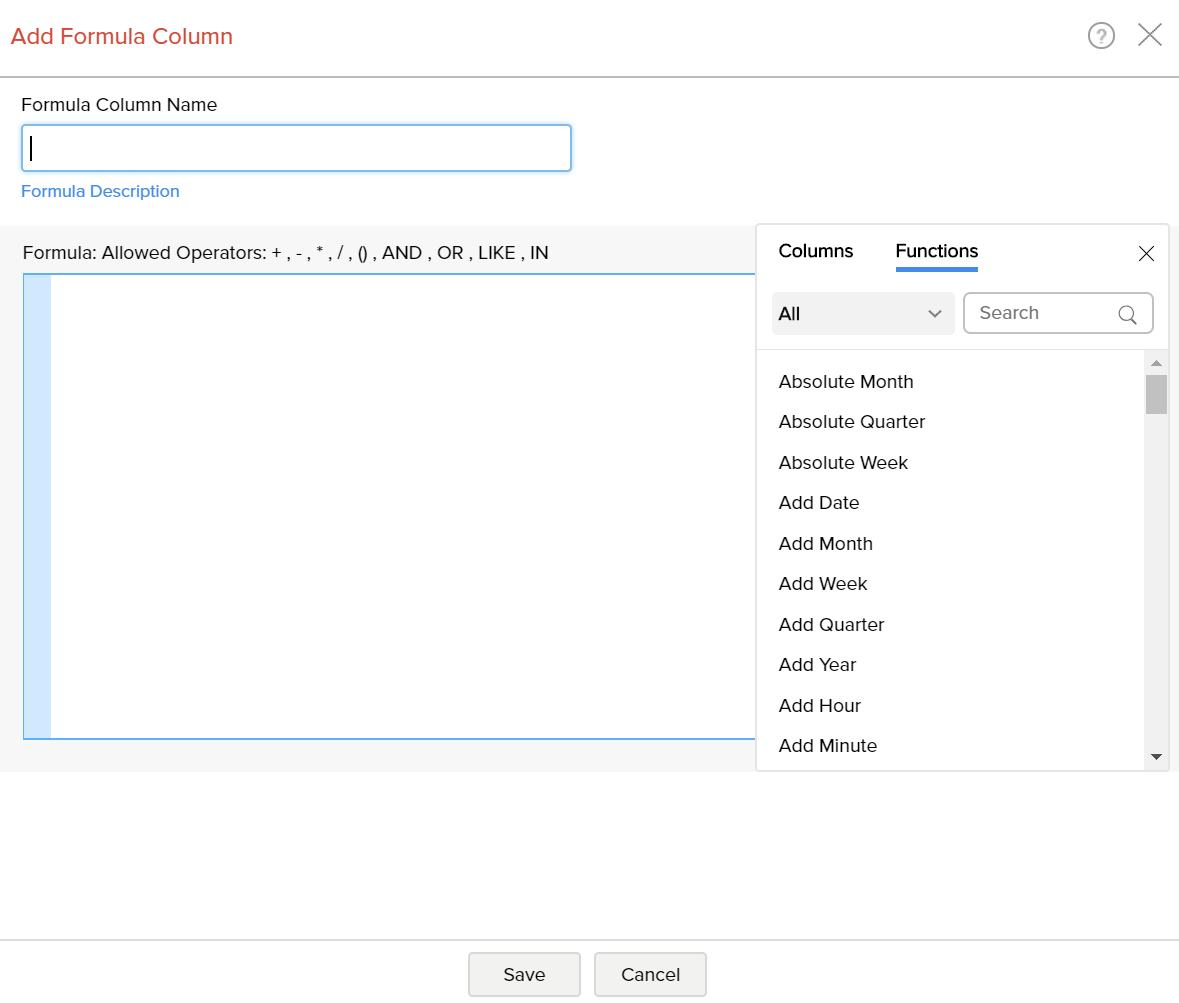• In the Formula Column dialog box, select the columns and functions that you want to insert from the list under the Columns and the Function boxes.

For example, to build a custom formula that adds particular number of days to a given date:

• Select the Add Date function under the Functions box. You will notice that adddate() syntax appears in the Formula text box.

• In the Formula text box, position the cursor in between the parenthesis, select the date column to which you wanted to add date using the Columns option.

• Enter the number of days (say 10) that you want to add separated by a comma.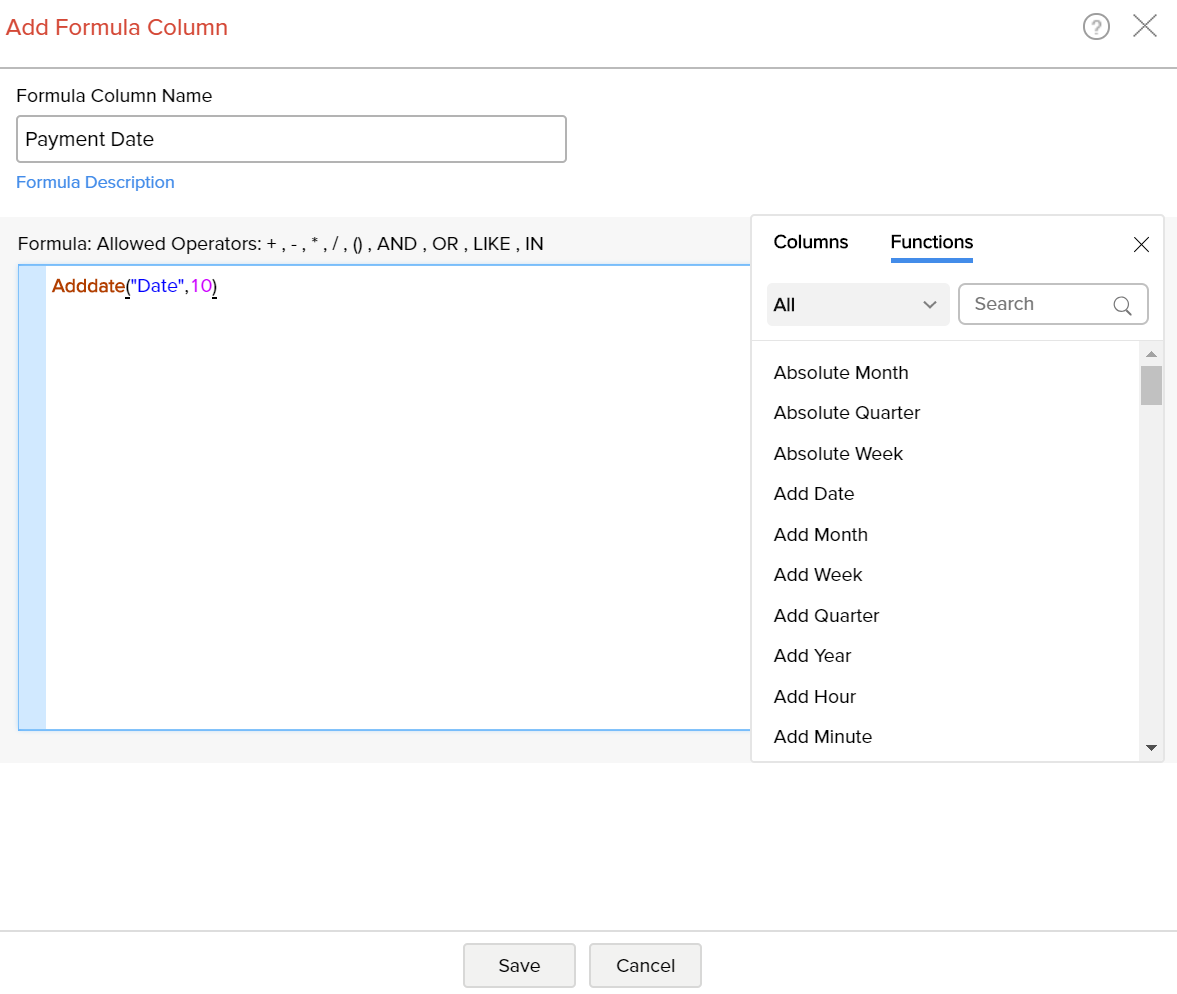To create a formula column with this expression, type column name in the Formula Column Name text box and then click OK. A new formula column will be created in your table as shown below.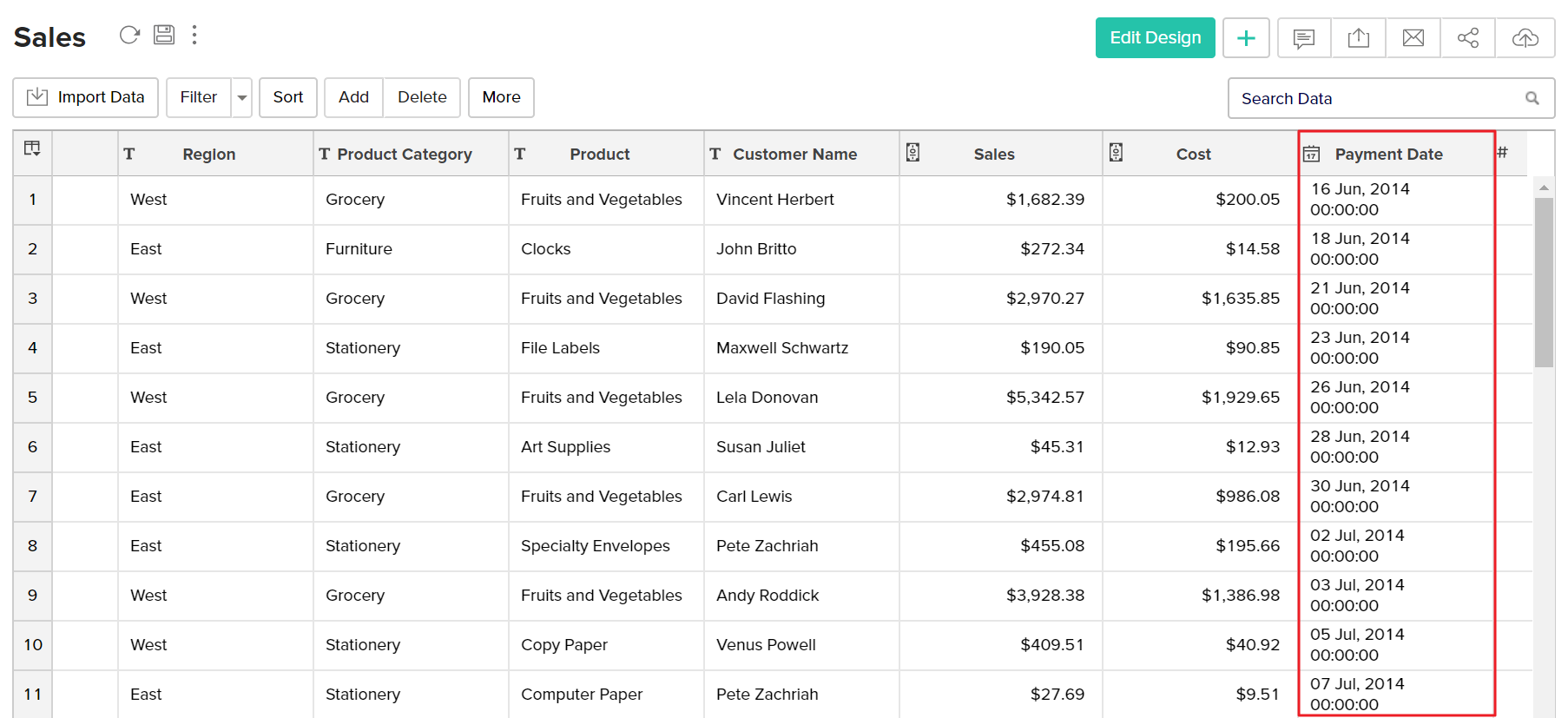You can edit the formula column any time by right clicking on the formula column listed in the table and clicking Edit Custom Formula in the popup menu. Also refer to View/Edit Formulas to know how you could view all the formulas defined on a table and handle edition or deletion of the same.

## Quick Add option for Formula Columns

ManageEngine Analytics Plus provides an easy and convenient way to add formula columns which are based on a limited set of widely used functions. You can find this quick add option as follow.

• Select a column based on which you want to add the formula column.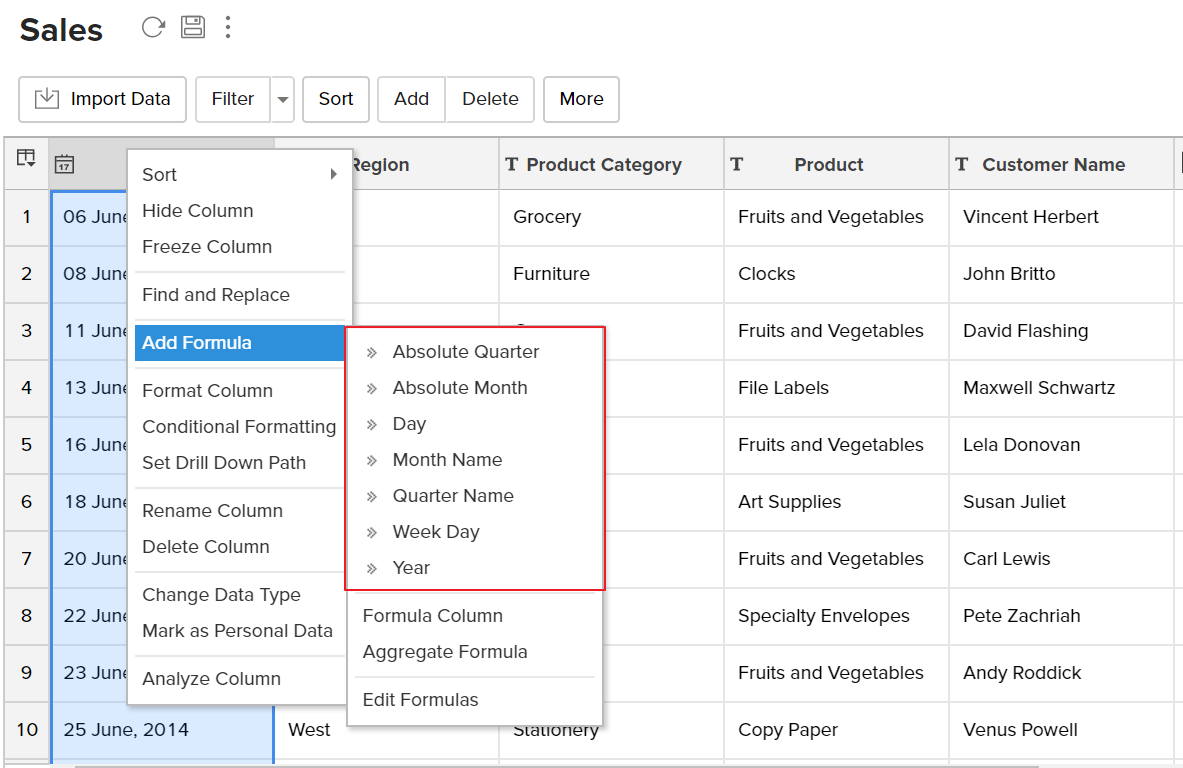• ManageEngine Analytics Plus automatically identifies the data type of the column selected and displays a list of widely used pre-defined formulas which are applicable to that as shown in the screenshot below.

• On selecting the required formula from the list, ManageEngine Analytics Plus applies the formula on the corresponding column and adds a new formula column, at the right end of the table.

## In-built functions provided by ManageEngine Analytics Plus

ManageEngine Analytics Plus provides you with a variety of in-built functions which are predefined mathematical formulas designed to perform specific well-known calculations easily. The following table gives a list of inbuilt functions provided by ManageEngine Analytics Plus which can be used to create any formula. The inbuilt formulas are categorized/grouped based on type of function.

#### Date Functions

FUNCTION

DESCRIPTION

EXAMPLE

Absolute Month - absmonth(date_column)

This function will return month and year from a given date value with the format (Month, yyyy).

absmonth('2011/8/7') = August, 2011

Absolute Quarter - absquarter(date_column)

This function will return Quarter and year from a given date value with the format (Quarter, yyyy).

absquarter('2011/8/7') = Q3, 2011

This function will add the specified number of days(num_of_days) to the given date value.

Returns the day by adding the time to the given date column.

('2002/02/21 18:23:26', '01:20:10') = 2002/02/21 19:43:36

Current Date - currentdate()

This function will return the current date of the computer or server.

currentdate() = 15 Sep, 2011 10:06:18

Date and Time Dif - dateand

timediff(Unit, From Date, To Date[optional]

This function will return the date and time difference between two date columns based on the units specified. The supported units are SECOND, MINUTE, HOUR, DAY, WEEK, MONTH, QUARTER, YEAR.

dateandtimediff(DAY, '2015-01-01', '2015-05-01')=120

The above example returns the difference between the given dates in Days.

Date Diff - datediff(date_column1, date_column2)

This function will return the difference between the two given date columns.

datediff('2011/8/11','2010/9/11') = 334

Day - day(date_column)

This function will return the day of the given date value.

day( '2011/9/9') = 9

Day of Week - dayofweek(date_column)

This function will return the number of the day of the week of the given date value (Sunday = 1, Monday = 2,...).

dayofweek('2011/9/9') = 6

Day of Year - dayofyear(date_column)

This function will return the number of the day of the year of the given date value (0 through 365).

dayofyear('2011/9/2') = 245

From Unixtime - fromunixtime(seconds)

This function returns the unix time for the given seconds value.

fromunixtime('1000') = 1970/01/01 05:46:40

Hour - hour(date_column)

This function returns the hour of the given date value.

hour('2011/8/7 10:35:23') = 10

Last Day - lastday(date_column)

This function will return the last day of the month for the given date value.

lastday('2011/9/7') = 2011/9/30

Make Date - makedate(year,num_of_days)

This function returns the date value for the given year and number of the day value (0 through 365)

makedate('2011','23') = 2011/1/23

Minute - minute(date_column)

This function returns the minutes of the given date value.

minute('2011/8/7 10:35:23') = 35

Modified Time -modifiedtime()

This function returns the created time of the record (if the record is newly added) or the last modified time of the record. When you apply this function, initially it will return the time at which the formula has been created. Subsequently it will return only the modified time of the record.

Month - month(date_column)

This function returns the name of the month of the given date value.

month('2011/9/7') = September

Month Num - monthnum(date_column)

This function returns the number of the month of the given date value.

monthnum('2011/9/7') = 9

Quarter - quarter(date_column)

This function returns the quarter of the given date value.

quarter('2011/8/7') = Q3

Second - second(date_column)

This function returns the seconds of the given date/time value.

second('2011/9/7 10:35:23') = 23

Sub Date - subdate(date_column,num_of_days)

This function returns the date by subtracting the number of days(num_of_days) from the given date value.

subdate('2011/9/15','6') = 1990/9/9

Sub Time - subtime(date_column,time)

This function returns the date by subtracting the time from the given date with time value.

subtime('2011/02/21 18:23:26','01:20:10') = 2011/02/21 17:03:16

Week Day - weekday(date_column)

This function returns weekday name (Sunday, Monday...) of the given date value.

weekday('2011/9/14') = Wednesday

Year - year(date_column)

This function returns year from the given date value.

year('2011/9/7') = 2011

#### Numeric Functions

FUNCTIONDESCRIPTIONEXAMPLE

Abs - abs(numeric_column)

This function returns the absolute value (number without sign) of the 'numeric_column'

pi() = 3.14159265358979

Acos - acos(numeric_column)

This function returns the arc cosine value of the specified 'numeric_column'. Returns NULL if the 'numeric_column' is not in the range-1 to 1.

pow(2,3) = 8

Asin - asin(numeric_column)

This function returns the arc sine value of the specified 'numeric_column'. Returns NULL if the 'numeric_column' is not in the range-1 to 1.

rand() = 0.282164005825449

Atan - atan(numeric_column)

This function returns the arc tangent value of the specified 'numeric_column'.

atan(2) = 1.107149

Atan2 - atan2(numeric_column1, numeric_column2)

This function returns the arc tangent of the specified columns 'numeric_column1' / 'numeric_column2

atan2(0.8, 0.6) = 0.927295

Ceil - ceil(numeric_column)

This functions rounds the 'numeric_column' to the nearest integer which is greater than the 'numeric_column'

ceil(11.56) = 12

Cos - cos(numeric_column)

This function returns the cosine value of the specified 'numeric_column'

cos(0) = 1

Cot - cot(numeric_column)

This function returns the cotangent value of the specified 'numeric_column'

cot(0.25) = 3.916317

Degrees - degrees(numeric_column)

This function returns the angle in Degrees equivalent to the given Radians

degrees(1) = 57.2957795

Exp - exp(numeric_column)

This function returns the exponential value of the 'numeric_column'

exp(2) = 7.389056

Floor - floor(numeric_column)

Rounds the 'numeric_column' to the nearest integer which is less than the 'numeric_column'

floor(11.56) = 11

Greatest - greatest(numeric_column,..., numeric_column)Gives the greatest of the given arguments.greatest(10,20,5) = 20
Least - least(numeric_column,..., numeric_column)Gives the least of the given arguments.least(10,20,5) = 5
Ln - ln(numeric_column)This function returns the natural logarithm of the specified 'numeric_column'ln(5) = 1.609438
Log10 - log10(numeric_column)This function returns the logarithm to the base-10 of the specified 'numeric_column'log10(3) = 0.477121
Log2 - log2(numeric_column)This function returns the logarithm to the base-2 of the 'numeric_columnlog2(32) = 5
Mod - mod(numeric_column1, numeric_column2)Returns the remainder of the 'numeric_column1' divided by 'numeric_column2'mod(10,3) = 1
Pi - pi()This function returns the numeric value of the pi.pi() = 3.14159265358979
Power - pow(numeric_column1, numeric_column2)This function returns the value of 'numeric_column1' raised to the power of 'numeric_column2'pow(2,3) = 8
Random - rand()Returns a random value between 0 and 1.rand() = 0.9233482386203
Round - round(numeric_column)Returns the rounded integer value of the 'numeric_column'.round(10.67) = 11
Sign - sign(numeric_column)Returns-1, 0, or 1, if the 'numeric_column' is negative, zero, or positive.sign(-23) =-1
Sin - sin(numeric_column)Returns the sine value of the 'numeric_column'.sin(0) = 0
Square - square(numeric_column)Returns the square of the specified 'numeric_column'.square(10) = 100
Square Root - sqrt(numeric_column)Returns the square root of the specified 'numeric_column'.sqrt(16) = 4
Tan - tan(numeric_column)Returns the tangent value of the specified 'numeric_column'.tan(0.5) = 0.546302

#### String Functions

FUNCTIONDESCRIPTIONEXAMPLE
Concat - concat(string_column,...,string_column)Returns the concatenated string of the given arguments. If any one of the argument is null, it returns null.concat('abcd','ef','db') = abcdefdbd
Concat_WS - concat_ws(separator,string_column1
,....,string_columnN)
Returns the concatenated string of the given arguments separated by the given separator. If the separator is null, it returns null.concat_ws('-','abcd','ef','db') = abcd-ef-db
Insert - insert(string_column, start_pos, len, new_string)Returns the string 'string_column', with the substring beginning at position 'start_pos' and 'len' characters long replaced by the string 'new_string'. 'start_pos' should be greater than 0. When len is zero, the 'new_string' is inserted previous to the position 'start_pos'.insert('abcddb', 3, 2, 'efgh') = abefghdb
Index of - indexof(string_column, sub_string)Returns the index of the first occurrence of the string 'sub_string' in string 'string_column'.indexof('abcddb','db') = 5
Left - left(string_column, len)Returns the 'len' number of characters from the left-hand side of the string 'string_column'.left('abcdef',3) = abc
Length - length(string_column)Returns the character length of the string.length('abcddb') = 6
Lowercase - lowercase(string_column)Returns the string 'string_column' with all characters changed to lowercase.lowercase('AbCD') = abcd
Locate - locate(sub_string, string_column, start_pos)cReturns the index of the first occurrence of the string 'sub_string' in string 'string_column' starting at the position 'start_pos'.locate('db','zohodbdb',6) = 7
Lpad - lpad(string_column, len, pad_string)Returns the string 'string_column', left-padded to a length of 'len' characters with the string 'pad_string'. If length of the string 'string_column' is greater than 'len', then the first 'len' characters of 'string_column' is returned.lpad('DB',5,'a') = aaaDB
Ltrim - ltrim(string_column)Returns the string 'string_column' with leading spaces removed.ltrim(' abcd') = abcd
Repeat - repeat(string_column,count)repeat('Abcd',3) = 'AbcdAbcdAbcd'
Replace - replace(string_column, from_string, to_string)Returns the string with all occurrences of the string 'from_str' replaced by the string 'to_str'replace('abcdac','ac','db') = abcddb
Reverse - reverse(string_column)Returns the reverse string of 'string_column'.reverse('abcd') = dcba
Right - right(string_column, len)Returns the 'len' number of characters from the right-hand side of the string 'string_column'right('abcdef',4) = cdef
Rpad - rpad(string_column, len, pad_string)Returns the string 'string_column', right-padded to a length of 'len' characters with the string 'pad_string'. If length of the string 'string_column' is greater than 'len', then the first 'len' characters of 'string_column' is returnedrpad('DB',5,'a') = DBaaa
Rtrim - rtrim(string_column)Returns the string 'string_column' with trailing spaces removed.rtrim('abcd ') = abcd
Strcmp - strcmp(string_column1, string_column2)Returns-1 if the 'string_column1' is smaller than the 'string_column2', 0 if the two strings are same, and 1 if the 'string_column1' is greater than the 'string_column2'.strcmp('abcd', 'abcde') =-1
Substring - substring(string_column, start_pos, string_len)The substring() method extracts the characters from a string, between two specified indices, and returns the new sub string.substring('abcddb', 1, 4) = abcd
Trim - trim(string_column)Returns the string with all spaces removed in prefix and suffix of the string.trim(' abcd ') = abcd
Uppercase - uppercase(string_column) &nbsp;Returns the string 'string_column' with all characters changed to uppercase.uppercase('abcD') = ABCD

#### Statistical Functions

FUNCTIONDESCRIPTION
Mean - mean(numeric_column)Returns the mean value of the 'numeric_column'
Median - (numeric_column)Returns the middle value in the 'numeric_column'
Mode - mode(numeric_column)Returns the most common value in the 'numeric_column

#### Logical Functions

FUNCTIONDESCRIPTIONEXAMPLE
IF - if(expr1,expr2,expr3)Returns expr2 if expr1 is true else it returns expr3if(5> 10,100,50) = 50
Ifnull - ifnull(expr1,expr2)Returns expr1 if expr1 is not null, else it return expr2ifnull(null,10) = 10
isnull( ) - isnull(expr1)Returns 1 if expr1 is null, else it returns 0.isnull(null)- 1

#### General Functions

FUNCTIONDESCRIPTION EXAMPLE
Coalesce - coalesce(null,null,1,...)Returns 1 if expr1 is null, else it returns 0.coalesce(null,null,4) = 4

## Creating Formulas Over Formula Column

Formula columns are similar to other columns of the table. You can make use of existing formula columns while creating a new formula column, as you do with other columns of the table. Reusing existing formulas column to create new formula columns helps you create powerful formula combination, as well as eases in maintenance of these formula structure in your workspace. Refer to the above section to know how to create formula column.

## Creating Reports Using Custom Formula Column

Custom Formula columns can be used while creating reports as you would use a column in a table. When you create a new report over the table, in which you have added the custom formula column, all these formula columns will be listed in the Columns list pane on the left, which can be drag and drop to create the report.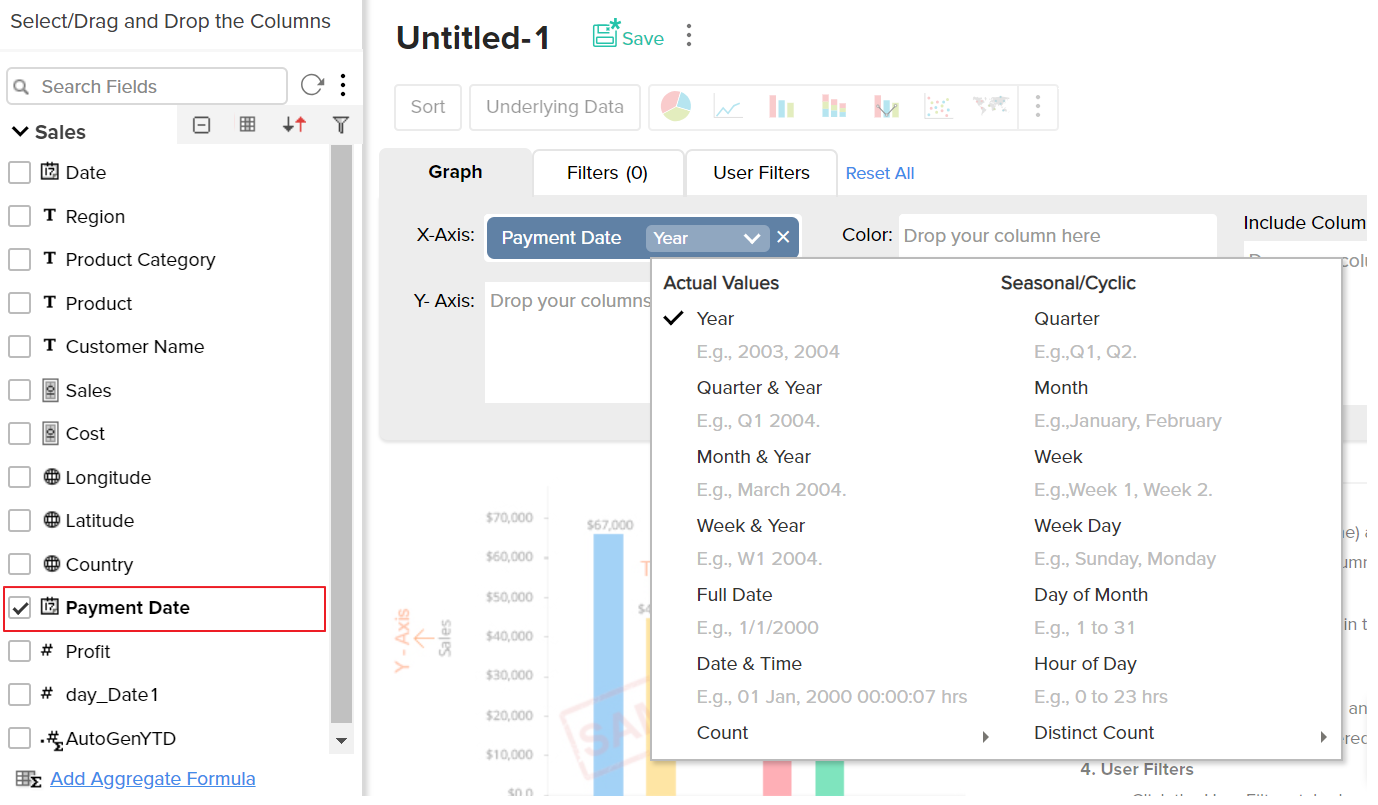You can apply functions on the formula column, as you do for other columns, using the combo box that is displayed over the column when dropped in the design area of the report.

## Sharing Custom Formulas

Once a Custom Formula column is created, it behaves like any other column in a table. When you share a table, all the existing custom formula columns will also be shared to the user.

Note:

• The shared user will not be able to edit, delete or format the shared formulas. Also the shared user will not be able to add his own formula column over the shared table, unless the shared user is a Workspace Owner.

## Aggregate Formulas

In reporting the most common data analysis that we do is to apply aggregate functions over the data (like SUM, AVG, COUNT etc.,) and analyze the same. The important aspect to be taken care in such calculations is to compute the aggregation in the context in which the function is applied to provide accurate results. Aggregate Formulas in ManageEngine Analytics Plus is meant to address this need.

Aggregate Formulas are formulas that you define in ManageEngine Analytics Plus which uses at least one aggregate function (SUM, AVG..) in the calculation. The output of such calculations is always a numeric value (metric). Aggregate formulas are mainly used in creating powerful reports. These formulas can be used in Charts, Pivot Tables and Summary View report types in ManageEngine Analytics Plus .

• The result of an Aggregate Formula is always a numeric value.
• Aggregate Formulas will not be added as another column in the base, it is associated with, like Custom Formulas. But it will be available for report creation when you create a report over the table (listed as part of the columns list).
• The value of an aggregate formula will be computed for each data point that is displayed in the report in which it is used (i.e., the result is not just a single value). This makes it very powerful.
• Aggregate formula can be constructed in a similar way as that of a Custom Formula.

ManageEngine Analytics Plus offers a range of in-built aggregate functions which can be used as part of the Aggregate Formulas. You can construct the formulas using these in-built aggregate functions combining it with the basic arithmetic operators like +, -, / and *.

## Creating Aggregate Formulas

To create an aggregate formula:

• Select Add > Aggregate Formula option from the toolbar as shown below.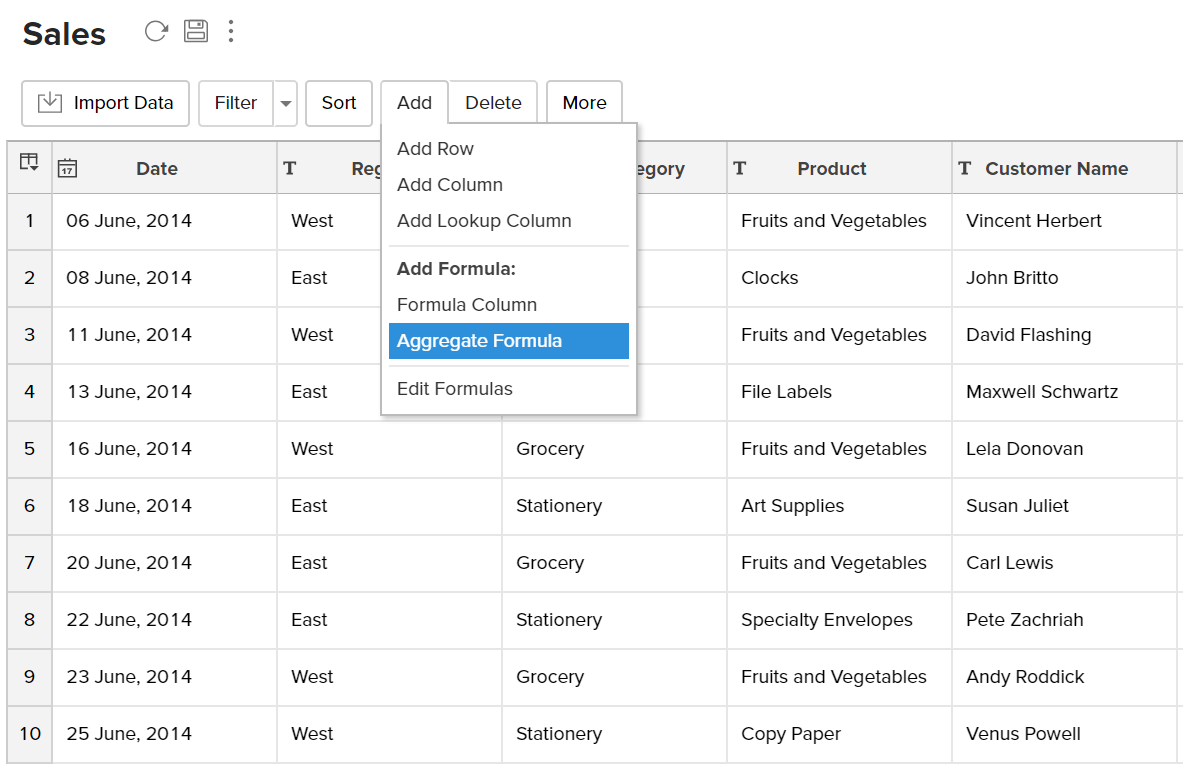• The Add Aggregate Formula dialog box will open as shown below. This dialog box provides easy access to the names of the columns in your table, as well as to all the in-built functions that are available for you to create the formula. A brief description of the function with syntax and an example will be displayed at the bottom of the dialog box when you hover your mouse over a function.

• In the Formula Column Name text box, type the name that you want to give to the formula.

• Select the data type of the aggregate result of the formula, using the DataType drop down menu. The data type should only be a Numeric value, hence the possible types are Number, Positive Number, Decimal Number, Currency and Percent.

• In the Formula text area provide the actual formula. You can select the columns and functions that you want to insert into the formula from the list from the Columns and the Function boxes.

Note: You can also insert columns from related tables joined through Lookup Column. The drop-down lists all the parent table associated with the base table. Select the required table, the columns in this table will be listed below. You can insert this in the formula by clicking.

• For example, to apply an aggregate formula to calculate % Profit the formula could be something like (SUM("SALE")- SUM("COST"))/SUM("COST")*100 as shown in the screenshot below.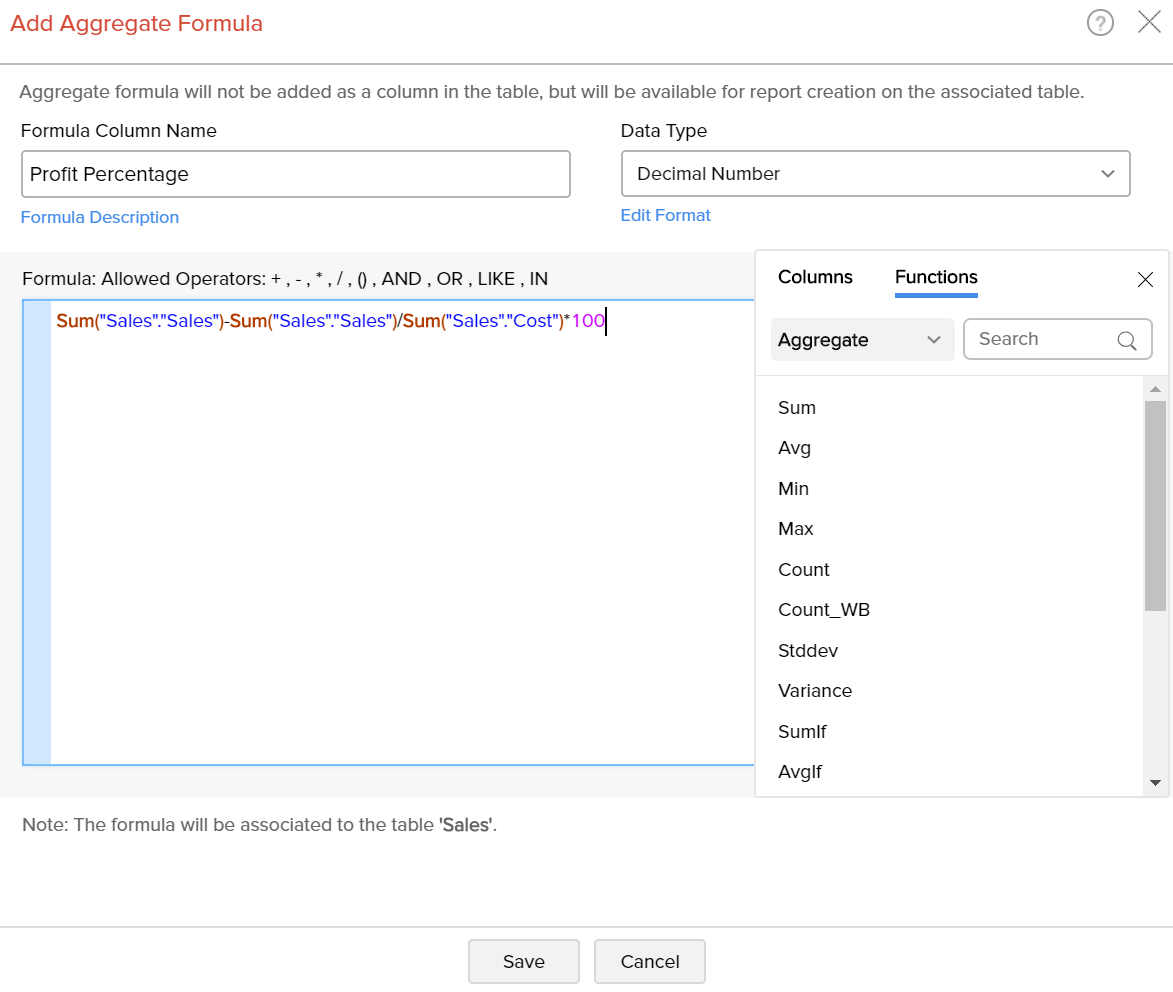• Click OK.

The result of Aggregate Formulas will not be added as a column in the base table. It will be listed in the column panel while creating reports. You can access (view) or edit it by clicking Add > Edit Formulas in the toolbar. Viewing & Editing Formulas is discussed in the next section.

## In-built Aggregate Functions

While you create an aggregate formula you can use a wide range of powerful in-built functions that are supported by ManageEngine Analytics Plus . You can use the in-built functions, that are applicable while creating custom formulas, even when you create an Aggregate formula, provided it meets the required conditions for an Aggregate formula. There are also specific functions which are more relevant & applicable for creating Aggregate formulas. The following table describes these functions:

 FUNCTION DESCRIPTION Sum - sum(numeric_column) Returns the sum of the 'numeric_column' Avg - avg(numeric_column) Returns the average of the 'numeric_column' Min - Min(numberic_column) Returns the minimum of the 'numeric column' Max - max(numeric_column) Returns the maximum of the 'numeric_column' Count - count(column) Returns the number of rows (values) in the given column Stddev - stddev(numeric_column) Returns the standard deviation of the 'numeric_column' Variance - variance(numeric_column) Returns the variance of the 'numeric_column' Distinct Count - count(distinct(column)) Returns the distinct number of values (rows) in the given column. SumIf - sumif(condition, expr1, expr2) Returns the sum of expr1, if condition is true. Else it will return the sum of expr 2. The expr1 and the expr2 can be an expression or just a numeric column. The expr2 is an optional argument. Example: sumif(Region = 'East, Sales, 0) will return the sum of values in the sales column for all the records which matches the value East in the Region column. Else it will return 0. AvgIf - avgif(condition, expr1, expr2) Returns the average of expr1, if condition is true. Else it will return the average of expr 2. The expr1 and the expr2 can be an expression or just a numeric column. The expr2 is an optional argument. Example: avgif(Region = 'East, Sales, 0) will return the average of values in the sales column for all the records which matches the value East in the Region column. Else it will return 0. CountIf- countif(condition) Returns the number of rows (records) that satisfies the condition. Example: countif(Region='East') will return the number of rows (records) in the table which matches the value East in the Region column. YTD -YTD(AggExpr,Date_Col) Year-to-dateis a period, starting from the beginning of the current year and ends at the current day. Arguments:AggExpr - Should be a Aggregate expression (which uses aggregate functions like SUM, AVG, ... ) for which the Year to date value will be calculatedDate_Col - Should be a date column based on which Year to Date will be calculated.Example: YTD(Sum(sales), OrderDate) will return the Sum of sales for the current Year till current day, based on date column 'OrderDat QTD - QTD(AggExpr,Date_Col) Quarter-to-dateis a period, starting from the beginning of the current quarter and ends at the current day. Arguments:AggExpr - Should be a Aggregate expression (which uses aggregate functions like SUM, AVG, ... ) for which the Quarter to date value will be calculatedDate_Col - Should be a date column based on which Quarter to Date will be calculated.Example: QTD(Sum(sales), OrderDate) will return the Sum of sales for the current Quarter till current day, based on date column 'OrderDate'. MTD - MTD(AggExpr,Date_Col) Month-to-dateis a period, starting from the beginning of the current month and ends at the current day. Arguments:AggExpr - Should be a Aggregate expression (which uses aggregate functions like SUM, AVG, ... ) for which the Month to date value will be calculatedDate_Col - Should be a date column based on which Month to Date will be calculated.Example: MTD(Sum(sales), OrderDate) will return the Sum of sales for the current Month till current day, based on date column 'OrderDate'.

## Creating Aggregate Formula over existing Aggregate Formulas

ManageEngine Analytics Plus enables you to build aggregate formulas using existing aggregate formulas. Reusing existing formulas to create new aggregate formula helps you create powerful formula combination, as well as eases maintenance of these formula structure in your workspace.

When you create a new aggregate formula, existing formulas will be listed in the Click to Insert Columns box along with other columns. All the aggregate formulas will be indicated in brown with the symbol. You can insert these formulas into your new formula definition by clicking them, as you do for the other columns in the table.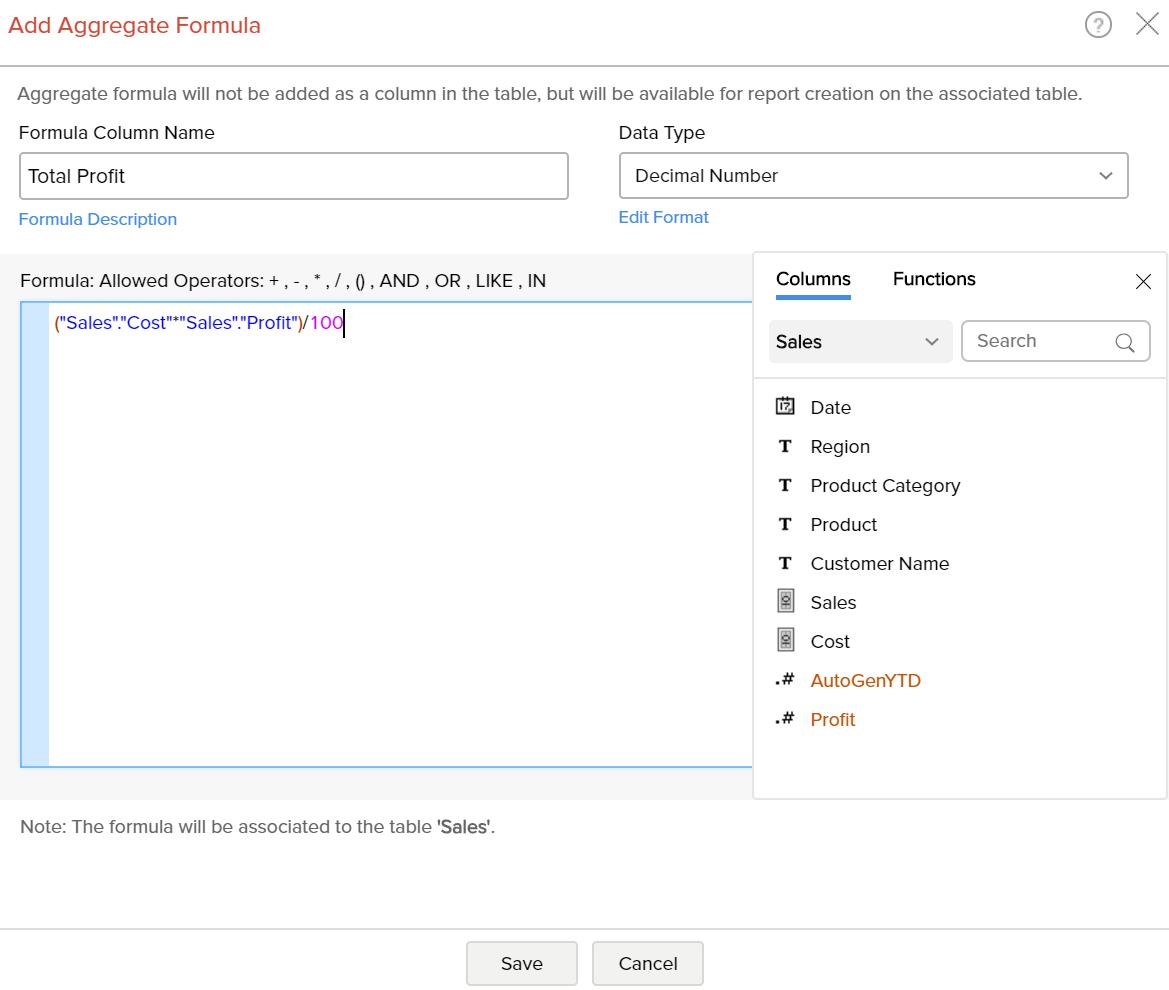For example, Let's assume that you have already calculated profit percentage and total cost and you want to calculate total profit gained. Then you can use the existing aggregate formulas to calculate total profit gained. The formula could be something like ("Total Cost" * "Profit") / 100.

## Creating Reports using Aggregate Formulas

Aggregate formulas can be used while creating all reports (charts, pivot tables and summary views) as you would use a column in a table. When you create a new report over the table, in which you have added the aggregate formula, all the formulas will be listed in the Columns list pane on the left, which can be drag & dropped to create the report.

The following screenshot shows the % Profit aggregate formula, that we added, listed in the columns list page. All the aggregate formulas will be indicated with an icon which contains the symbol.

From the Explorer tab of the workspace, select the table to which you want to add the aggregate formula.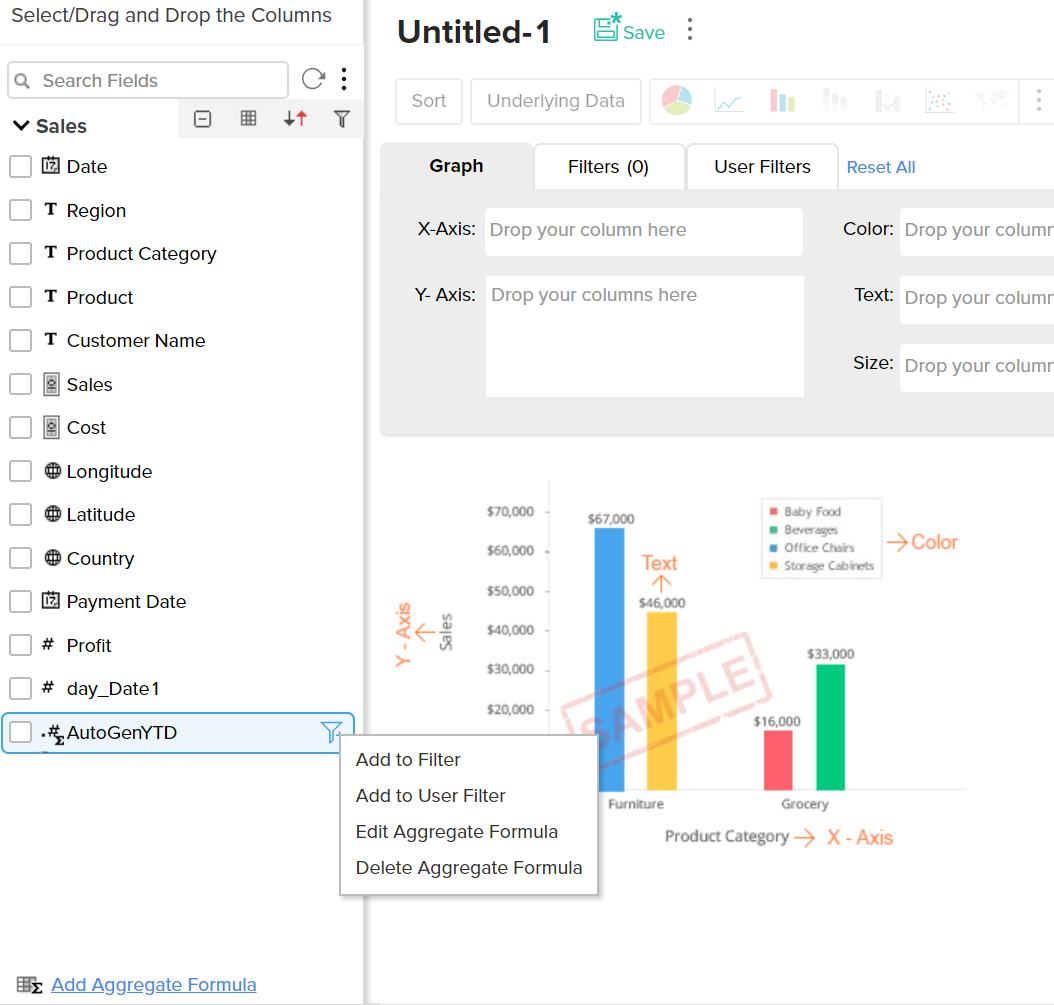Aggregate formulas can be used in the report as you use any other data columns in the table. The value of the aggregate formula will be computed for each data point that is displayed in the report in which it is used (i.e., the result is not just a single value). In the chart shown in the screenshot below, the percentage of profit (%Profit) is plotted against the Year. As you see, the value of the Profit percentage is calculated for each year and plotted in the graph.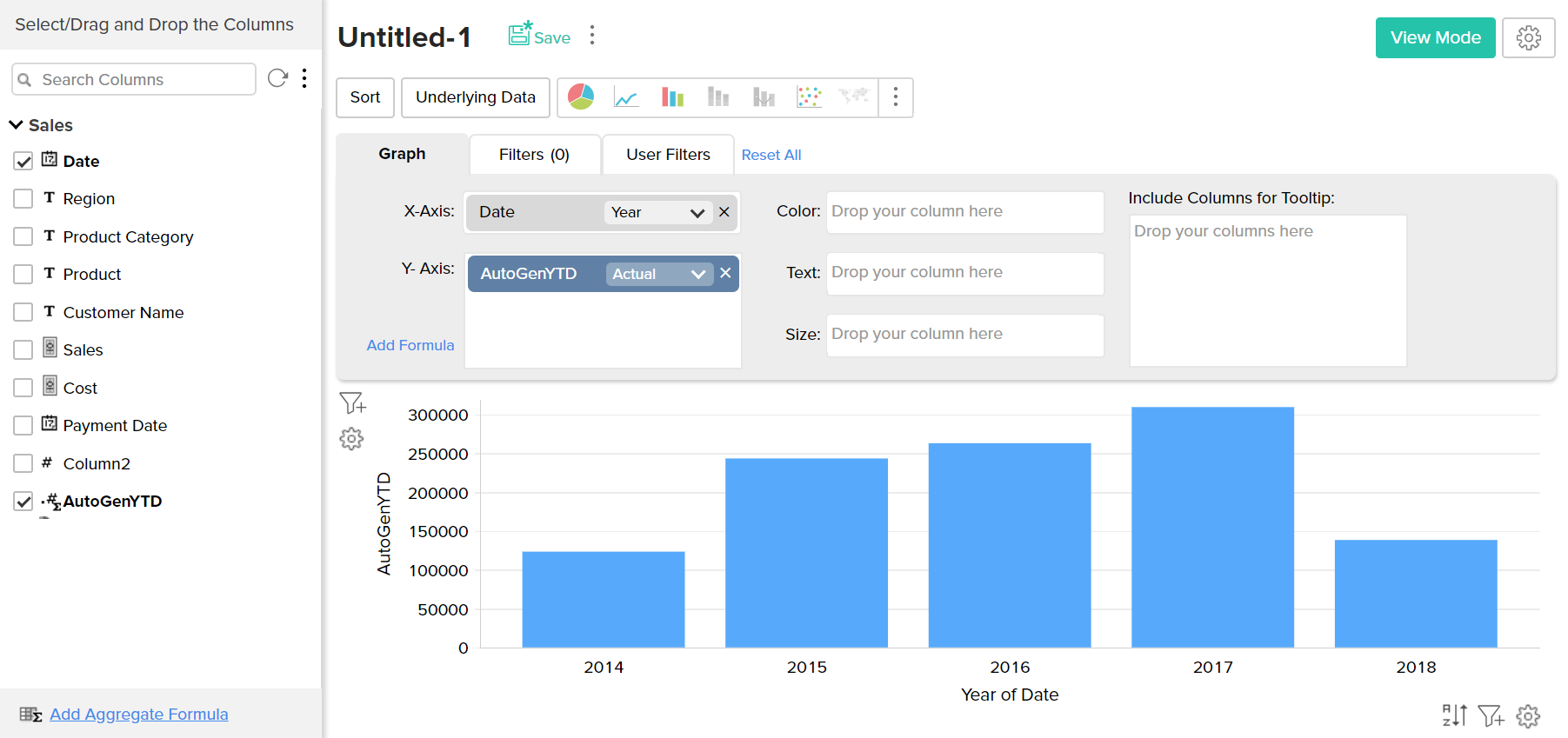When you use an Aggregate formula in a report, you will not be able to apply other functions on the formula (as you do for other columns). The function will always be selected as 'Actual' and cannot be modified.

## Sharing Aggregate Formulas

With respect to sharing and collaboration, Aggregate Formulas behave similar to how a column in a table behaves. When you share a table, all the aggregate formulas that are created over the table will be also shared to the user to whom you are sharing the table. Hence the shared user will be able to use these formulas while creating any report over the data table.

Note:

• The shared user will not be able to edit, delete or format the shared aggregate formula, but can just use it in report creation. Also the shared user will not be able to add his own Aggregate formulas over the shared table, unless the user is a Workspace Owner.

## View/Edit Formulas

ManageEngine Analytics Plus allows you to view, edit, format and delete all the formulas defined for a table through the Edit Formula option. You can view & edit both Custom Formulas and Aggregate Formulas using this option. To view/edit existing custom or aggregate formulas:

• Select the required table.

• Select Add > Edit Formulas option from the toolbar.

• The Edit Formula dialog box with a list of all the formulas associated to the table will open as shown below: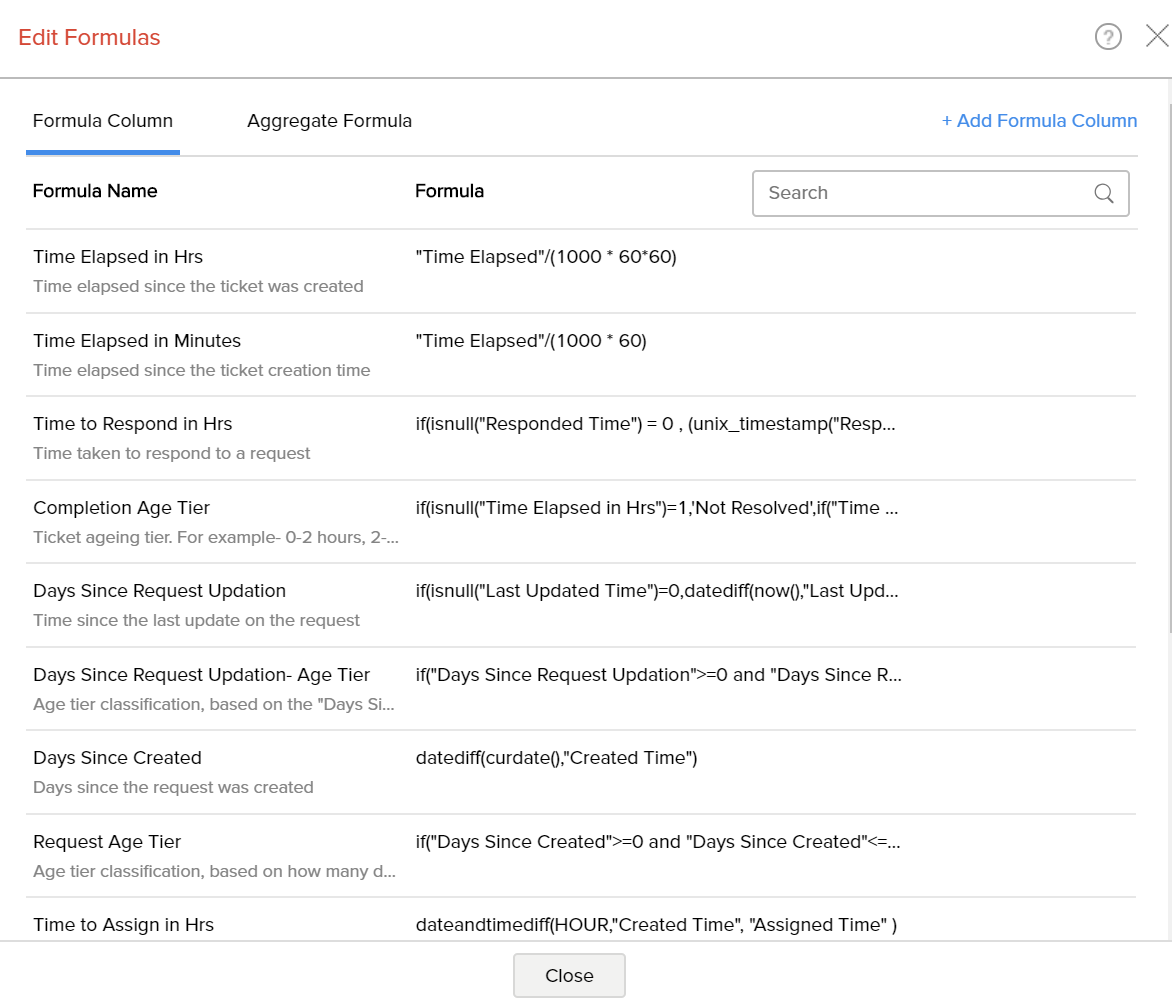On hovering over a specific formula, the EditRemove and Format options are available.

• Edit Formula: You can edit the constructed formula using the Edit link provided next to the corresponding formula. The Edit Formula/ Aggregate Formula (depending on the type of formula) dialog box will open, and you can modify the existing formula the same way you would when creating a new formula.
• Delete Formula: You can delete an existing Formula any time from the table using the Remove link provided next to the corresponding formula.
• Format Formula: You can change the format of the Formula result, like alignment, decimal places, date format, currency symbols etc., depending on its data type of the formula using the Format link provided. It's similar to how you format a data column. Refer here to know how to format a column.